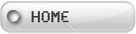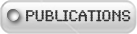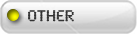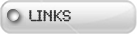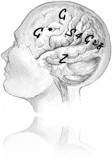## G, G*, S4Grz, Z, …

Here are programs for G, G*, S4Grz, Z and Z* (Z are the KD logics coming with the []p & <>p variant, but without the restriction to the Σ1 sentences). IL is for intuitionistic logic. gip, g*ip, ilip are G, G*, IL, working on infix presentation of formula. So (g '(->(bw p) (bw (bw p))) gives the same answer as (gip '(bw p -> bw bw p)). []p is represented by (bw p). (g A) gives NIL if the formula A is a theorem of g, meaning the list of counterexample is empty, otherwise it gives Kripke models invalidating the formula A. Just read those heavy answers as "not a theorem". But if you want to read the Kripke models just note that F\# represent the node of the tree of the accessibility relation on worlds, and that the worlds are represented by lists with the relevant sentences. G, G*, … Taken from my IRIDIA technical report 1994 (Conscience et Mécanisme).